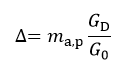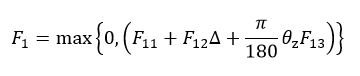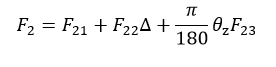This function computes the extraterrestrial irradiance outside of the earth’s atmosphere, based a Fourier series representation of the position of the sun (earth-sun distance).

## Inputs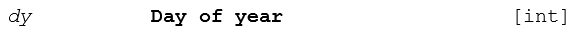## Outputs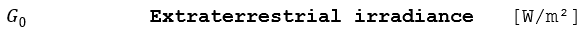## Algorithm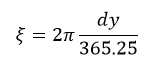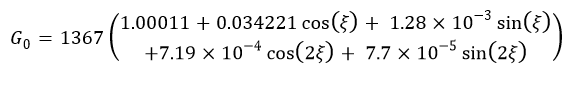## Reference

Bird, R., Hulstrom, R., A Simplified Clear Sky Model for Direct and Diffuse Insolation on Horizontal Surfaces, 1981
NREL SERI/TP-642-761

Spencer, J. W., Fourier Series Representation of the Position of the Sun, Vol. 2, 1971, p. 172.

# Clearness Index

This function computes the global horizontal clearness index with respect to the extraterrestrial irradiance on the
horizontal surface.

## Inputs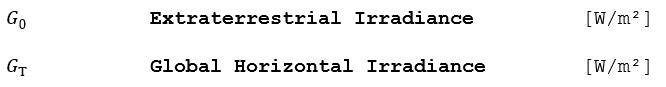## Algorithm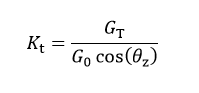## Reference

Bird, R., Hulstrom, R., A Simplified Clear Sky Model for Direct and Diffuse Insolation on Horizontal Surfaces, 1981
NREL SERI/TP-642-761

# Air Mass

This function empirically computes the non-pressure-corrected (sea level) and pressure-corrected (at altitude) air
masses as a function of the solar zenith angle.

## Inputs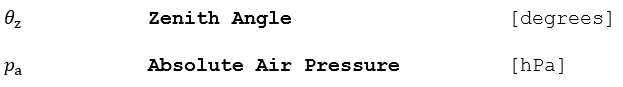## Outputs## Algorithm (Bird & Hulstrom Version)

Compute the sea-level air mass: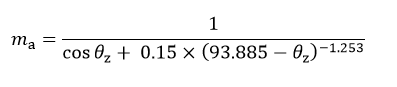## Algorithm (Kasten / Sandia Version)

Compute the sea-level air mass: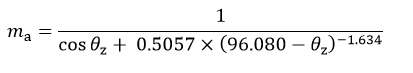## Algorithm (Common Pressure Correction and Filtering)

1.) Restrict the calculation of the air mass for zenith angles less than 89°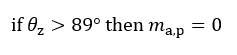2.) Reduce the air mass function by the actual pressure (a function of elevation or an input given in the weather
data):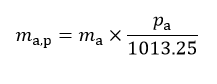## Reference

Bird, R., Hulstrom, R., A Simplified Clear Sky Model for Direct and Diffuse Insolation on Horizontal Surfaces, 1981
NREL SERI/TP-642-761

Kasten, F. Discussions on the Relative Air Mass, Light Research Technology 25, 129.

This function computes the direct normal irradiance from the global horizontal and diffuse irradiance, if no direct normal irradiance is available.

## Inputs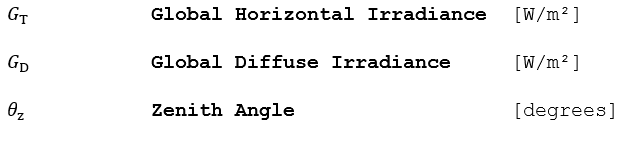## Outputs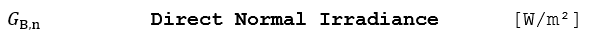## Algorithm (Bird & Hulstrom Version)

Compute the direct normal irradiance only if GT > GD else set it to 0, as follows: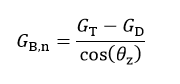PlantPredict implements three diffuse-direct decomposition models to compute the diffuse irradiance from the global horizontal irradiance if neither diffuse irradiance nor direct normal irradiance is available in the weather file. The choice of models is user-selectable:

# Reindl

This function computes the direct normal irradiance and the diffuse global irradiance from the global horizontal according to the Reindl correlation model, if no direct normal or diffuse irradiance is available. Three versions of the model are available in literature, as illustrated in Figure 6 :

1. Function of zenith angle, clearness index, air temperature, and relative humidity (blue).
2. Function of zenith angle and clearness index (red)
3. Function of clearness index only (green)

A minor modification to the published algorithm was made based on one year of hourly global and diffuse measurements at a utility-scale power plant. At very high clearness indices, the discontinuity between segments B and C of the piecewise regression model is too abrupt, leading to discontinuous irradiance profiles on even clear days.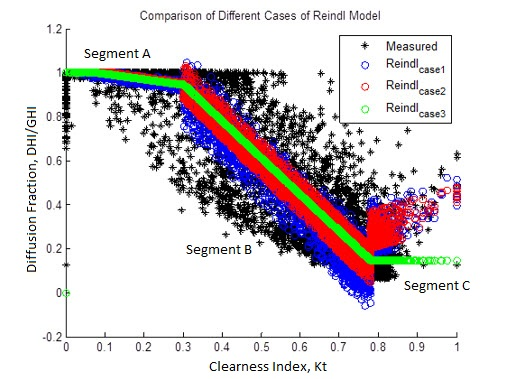### Figure 6. Reindl Diffuse-Direct Decomposition Model Compared to Measured Data

The discontinuity at Kt = 0.78 is problematic for clear sky days when there is little measured diffuse irradiance (black). An adjustment was made, as shown in Figure 7.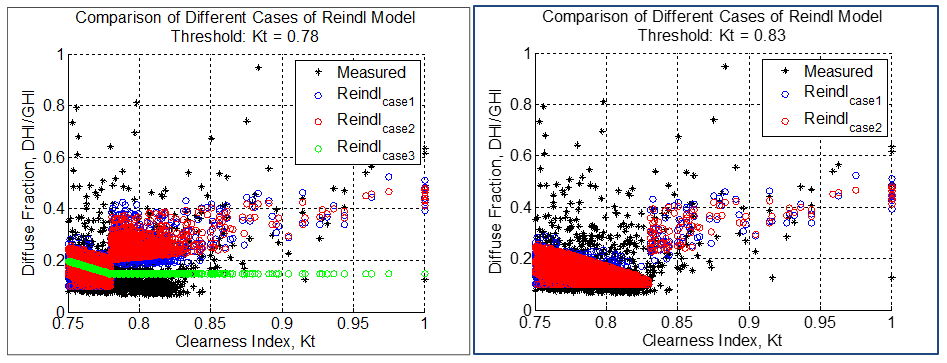### Figure 7. Modification of then Reindl Diffuse Correlation Model

This causes a large discontinuity in the errors of modeled DHI during these times. Based on this residual analysis it appears that the error in the Reindl model in sloped segment B is large up to a Kt of 0.83 – 0.84. As a result, the threshold between segments B and C was moved from 0.78 to 0.83.

## Outputs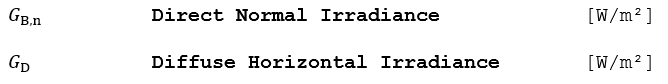## Algorithm

Only versions (1) and (2) of the algorithm (as described above) are implemented, as version (3) is not necessary since ambient temperature is required in all PlantPredict weather files. If Φ is available, version (1) is used; if Φ is missing, then PlantPredict automatically defaults to version (2).

1.) Find the piecewise correlation of the diffuse fraction if air temperature and relative humidity are known (fd, first equation), or unknown (f’d, second equation). If the diffuse fraction falls out of the allowed range, clamp the result at the boundary value. Also note that these equations use the solar zenith angle, not the solar elevation angle (as defined in the Reindl paper).

1. If 0 ≤ Kt ≤ 0.3: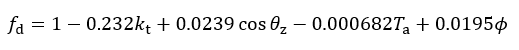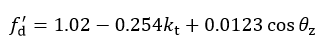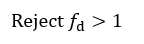1. If 0.3 < Kt < 0.83: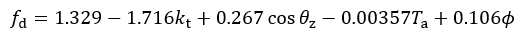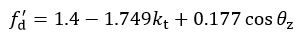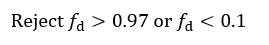1. If 0.83 ≤ Kt: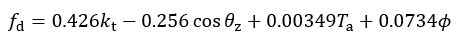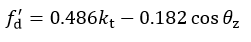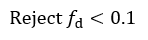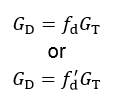3.) Compute the direct normal irradiance only if GT > GD, as follows and if θz < 89°: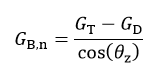Else, set G B,n to 0

## Reference

Reindl, D. T., Beckman, W. A., Duffie, J. A., Diffuse Fraction Corrections, Solar Energy Vol. 45, No. 1, pp. 1-7, 1990.

# Erbs Model

This routine estimates the direct normal and diffuse horizontal irradiance using the Erbs correlation.

## Outputs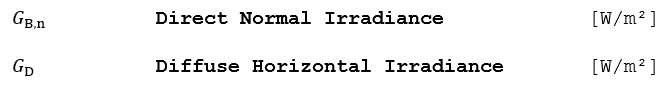## Algorithm

1.) Find the piece-wise correlation of the diffuse fraction fd :

1. If 0 ≤ Kt ≤ 0.22: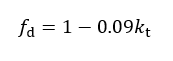1. 2.If 0.22 < Kt ≤ 0.80:1. If 0.80 < Kt: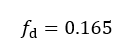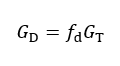3.) Compute the direct normal irradiance only if GT > GD, as follows and if θz < 89°: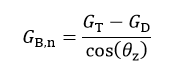## Reference

Erbs, et al, Estimation of the diffuse radiation fraction for hourly, daily and monthly-average global radiation. Solar Energy, 28:293-302, 1982.

# DISC Model

This routine implements the DISC correlation function, which is not included by itself as an option in PlantPredict; however, it is used by both the DIRINT decomposition and Hay transposition models.

## Algorithm

1.) Compute the clear-sky normal transmittance for all solar zenith angles.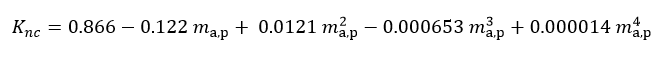2.) Compute coefficients a, b and c for the beam transmittance equation.

1. If Kt ≤ 0.60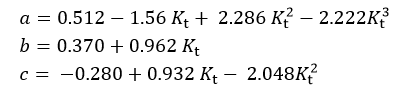1. If Kt > 0.60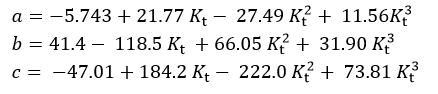3.) Compute the direct normal transmittance.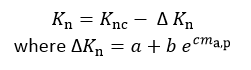4.) Compute the DIRINT direct normal irradiance.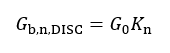5.) Set Gb,n,DISC to 0 if θZ > 87°, GT < 1, or Gb,n,DISC < 0

## Reference

Maxwell, E. L., A Quasi-Physical Model for Converting Hourly Global Horizontal to Direct Normal Insolation.

Technical Report No. SERI/TR-215-3087, Golden, CO: Solar Energy Research Institute, 1987.

# DIRINT Model

This routine determines direct normal irradiance using the DIRINT modification of the DISC model.

## Inputs## Outputs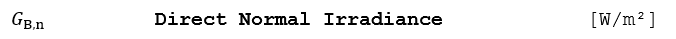## Algorithm

1.) Compute KT´.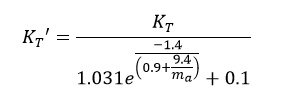2.) Generate ∆KT´.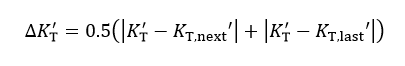Where KT,next´ is the next time series value of KT´ and K ´T is the previous time series value.

3.) Create KT´ bins.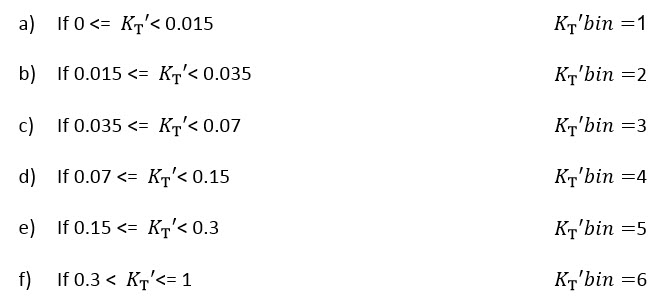4.) Create θZ bins.5.) Set perceptible water bin, Wbin, to 5, as this adjustment is currently not implemented in PlantPredict.

6.) Create ∆KT´ bins.7.) Perform 4-D lookup of DIRINT coefficients using KT´bin, θZ bins, ∆ KT´ bins, and Wbin to get DIRINTcoeff.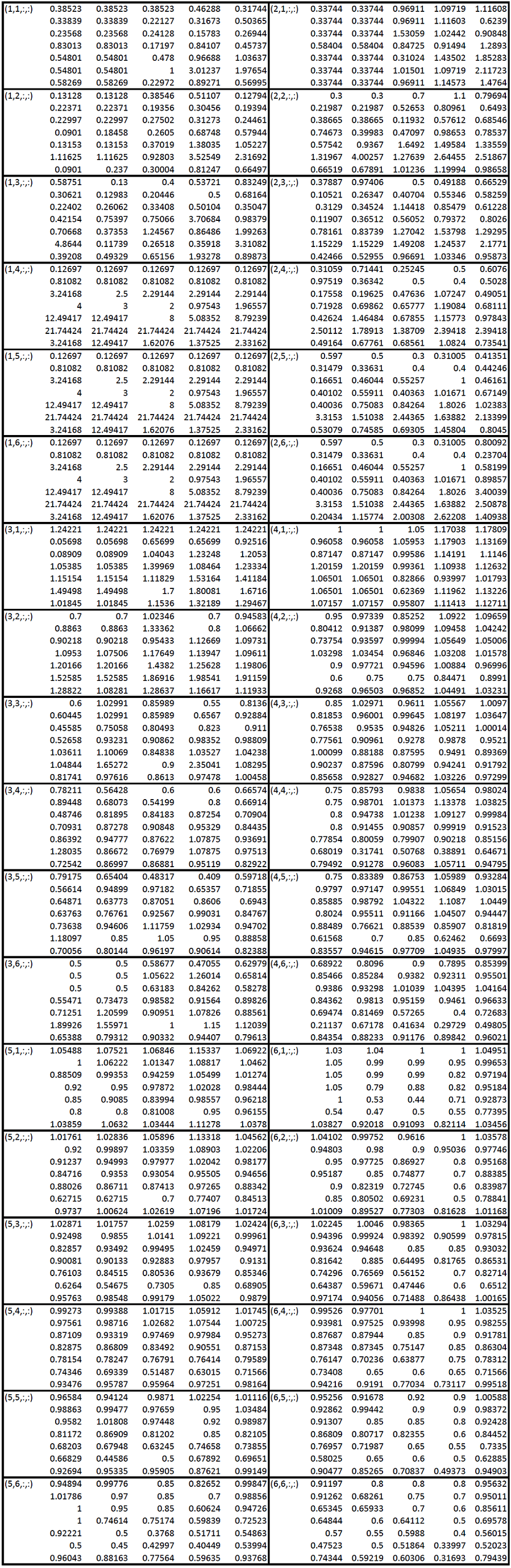8.) Calculate DIRINT direct normal irradiance.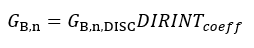## Reference

Perez, R., P. Ineichen, E. Maxwell, R. Seals and A. Zelenka, Dynamic Global-to-Direct Irradiance Conversion Models. ASHRAE Transactions-Research Series (1992), pp. 354-369.

Maxwell, E. L., A Quasi-Physical Model for Converting Hourly Global Horizontal to Direct Normal Insolation. Technical Report No. SERI/TR-215-3087, Golden, CO: Solar Energy Research Institute, 1987.

# Bird Sky Clear Model

This function computes the apparent shift in the solar elevation angle caused by atmospheric refraction.

Note that the output is a positive number; i.e.: near the horizon, the solar disk appears higher in the sky than its true position. The apparent elevation is the true (geometrical) elevation plus the correction factor.

## Outputs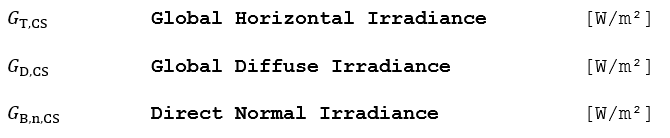## Algorithm

The current implementation assumes default values for the following parameters: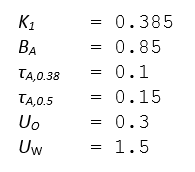## Reference

Bird, R., Hulstrom, R., A Simplified Clear Sky Model for Direct and Diffuse Insolation on Horizontal Surfaces, 1981 NREL SERI/TP-642-761

# Tilt Model

This function geometrically transposes the direct normal irradiance on an arbitrary tilted plane, and transposes the diffuse horizontal irradiance on the same tilted plane using a choice of user-selectable tilt models: Perez or Hay.

## Inputs## Outputs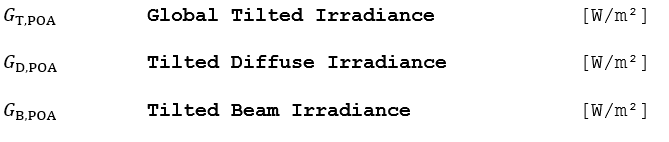# Perez Algorithm (Diffuse Sky)

1.) Find the factors that account for the respective angles of incidence of circumsolar radiation on the tilted and horizontal surfaces. The circumsolar radiation is considered to be from a point source.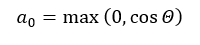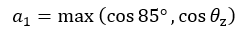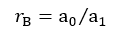2.) Compute the sky clearness as a function of the diffuse irradiance, direct normal irradiance, and zenith angle: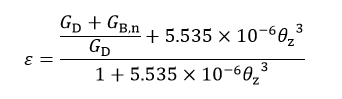3.) Find the brightness coefficients F1 and F2 from the lookup table in Figure 8 and the following equations: